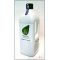##### The Japanese Bonsai specialist
Direct order Contact Help / Services Newsletter# Biogold vital bonsai treatment 2 ltr

Vital en deux litresref. : 5225

58,00

Available quantity : 8Order

###### Description

For healthy green leaves on indoor and outdoor bonsai use this treatment to give your trees a new lease of life. Quick and easy to use, refill a spray bottle and apply the fertiliser to deciduous trees, conifer vegetation and kusamono small plants.

2 litre can containing concentrated solution. Dilute one 30ml capful into 3 litres of water. In total, you can make 200 litres of fertiliser.

How to use:

Spray on foliage 1 to 2 times per month. Rapidly absorbed. If the plant is particularly weak you can spray it once or twice every 10 days.

Spray on the earth once a month.

Soak seeds for one or two hours in the solution before sowing. This will ward off fungal attacks and aid root development.

Our range of Biogold granules complement this product.

#spray 4.5 #treatment 3.9 #biogold 3.7 #bonsai 3.6 #this 3.4 #fertilizer 3 #fertiliser 3 #solution 2.8 #litres 2.6 #vital 2.5

Formule
(( ROUND((CHAR_LENGTH(b.article_nom)-CHAR_LENGTH(REPLACE(b.article_nom, 'spray', '')))/LENGTH('spray')) + ROUND((CHAR_LENGTH(b.article_description)-CHAR_LENGTH(REPLACE(b.article_description, 'spray', '')))/LENGTH('spray')) ) * 4.5) + (( ROUND((CHAR_LENGTH(b.article_nom)-CHAR_LENGTH(REPLACE(b.article_nom, 'treatment', '')))/LENGTH('treatment')) + ROUND((CHAR_LENGTH(b.article_description)-CHAR_LENGTH(REPLACE(b.article_description, 'treatment', '')))/LENGTH('treatment')) ) * 3.9) + (( ROUND((CHAR_LENGTH(b.article_nom)-CHAR_LENGTH(REPLACE(b.article_nom, 'biogold', '')))/LENGTH('biogold')) + ROUND((CHAR_LENGTH(b.article_description)-CHAR_LENGTH(REPLACE(b.article_description, 'biogold', '')))/LENGTH('biogold')) ) * 3.7) + (( ROUND((CHAR_LENGTH(b.article_nom)-CHAR_LENGTH(REPLACE(b.article_nom, 'bonsai', '')))/LENGTH('bonsai')) + ROUND((CHAR_LENGTH(b.article_description)-CHAR_LENGTH(REPLACE(b.article_description, 'bonsai', '')))/LENGTH('bonsai')) ) * 3.6) + (( ROUND((CHAR_LENGTH(b.article_nom)-CHAR_LENGTH(REPLACE(b.article_nom, 'this', '')))/LENGTH('this')) + ROUND((CHAR_LENGTH(b.article_description)-CHAR_LENGTH(REPLACE(b.article_description, 'this', '')))/LENGTH('this')) ) * 3.4) + (( ROUND((CHAR_LENGTH(b.article_nom)-CHAR_LENGTH(REPLACE(b.article_nom, 'fertiliser', '')))/LENGTH('fertiliser')) + ROUND((CHAR_LENGTH(b.article_description)-CHAR_LENGTH(REPLACE(b.article_description, 'fertiliser', '')))/LENGTH('fertiliser')) ) * 3) + (( ROUND((CHAR_LENGTH(b.article_nom)-CHAR_LENGTH(REPLACE(b.article_nom, 'solution', '')))/LENGTH('solution')) + ROUND((CHAR_LENGTH(b.article_description)-CHAR_LENGTH(REPLACE(b.article_description, 'solution', '')))/LENGTH('solution')) ) * 2.8) + (( ROUND((CHAR_LENGTH(b.article_nom)-CHAR_LENGTH(REPLACE(b.article_nom, 'litres', '')))/LENGTH('litres')) + ROUND((CHAR_LENGTH(b.article_description)-CHAR_LENGTH(REPLACE(b.article_description, 'litres', '')))/LENGTH('litres')) ) * 2.6) + (( ROUND((CHAR_LENGTH(b.article_nom)-CHAR_LENGTH(REPLACE(b.article_nom, 'vital', '')))/LENGTH('vital')) + ROUND((CHAR_LENGTH(b.article_description)-CHAR_LENGTH(REPLACE(b.article_description, 'vital', '')))/LENGTH('vital')) ) * 2.5) + (( ROUND((CHAR_LENGTH(b.article_nom)-CHAR_LENGTH(REPLACE(b.article_nom, 'month', '')))/LENGTH('month')) + ROUND((CHAR_LENGTH(b.article_description)-CHAR_LENGTH(REPLACE(b.article_description, 'month', '')))/LENGTH('month')) ) * 2.5)

## Secure payment## Delivery

Our logistic partners :